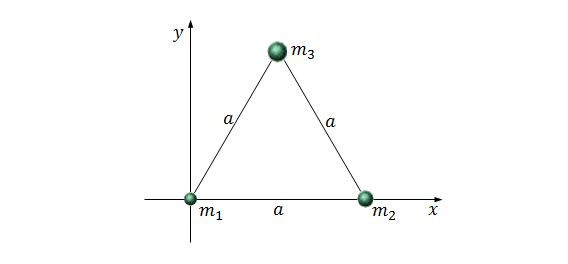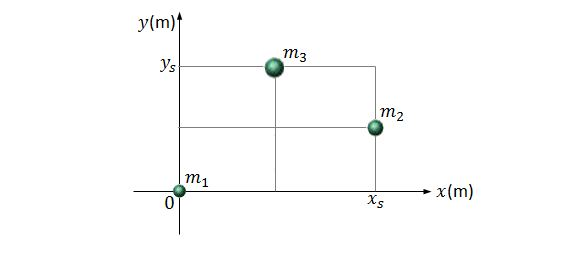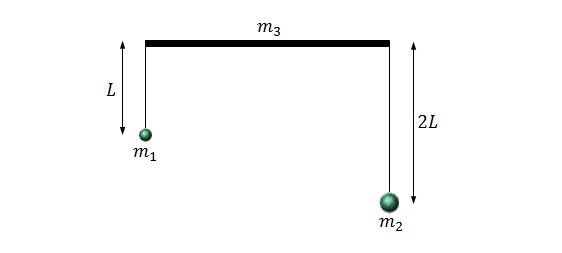Classical Mechanics

# Center of mass of a collection of pointsThree particles of respective masses $m_1=12.0\text{ kg},$ $m_2=25.0\text{ kg}$ and $m_3=38.0\text{ kg}$ form an equilateral triangle of side length $a=140\text{ cm}.$ If we locate $m_1$ at the origin on the $xy$-plane, and put $m_2$ to the right of $m_1$ on the $x$-axis, as shown in the above figure, what are the approximate coordinates of the center of mass of this system?

The value of $\sqrt{3}$ is $1.732.$Three particles are on the $xy$-plane, as shown in the above figure. The masses of the three particles are $m_1=2.0\text{ kg},$ $m_2=5.0\text{ kg}$ and $m_3=9.0\text{ kg}.$ If the scales on the axes are set by $x_s=4.0\text{ m}$ and $y_s=6.0\text{ m},$ what are the $xy$-coordinates of the system's center of mass?Consider a uniform bar of length $3L$ and mass $m_3=5m.$ Two balls are hanging on strings with negligible mass from the two ends of the bar, and their masses are $m_1=m$ and $m_2=3m.$ The lengths of the string on which the balls are hanging are $L$ and $2L,$ respectively, as shown in the above figure. What is the center of mass of this system relative to the midpoint of the bar?

Three particles $A,$ $B$ and $C$ are on the $xy$-plane. Their masses are $m_A=2.00\text{ kg},$ $m_B=4.00\text{ kg}$ and $m_C=3.00\text{ kg},$ and the coordinates of $A$ and $B$ are $(-1.40\text{ m},0.48\text{ m})$ and $(0.70\text{ m},-0.72\text{ m}),$ respectively. If the coordinates of the center of mass of the three-particle system is $(-0.50\text{ m},-0.70\text{ m}),$ what is the coordinates of particle $C ?$Three particles of respective masses $m_1=13.0\text{ kg},$ $m_2=29.0\text{ kg}$ and $m_3=37.0\text{ kg}$ form an equilateral triangle of side length $a=140\text{ cm}.$ If we locate $m_1$ at the origin on the $xy$-plane, and put $m_2$ to the right of $m_1$ on the $x$-axis, as shown in the above figure, what are the approximate coordinates of the center of mass of this system?

The value of $\sqrt{3}$ is $1.732.$

×